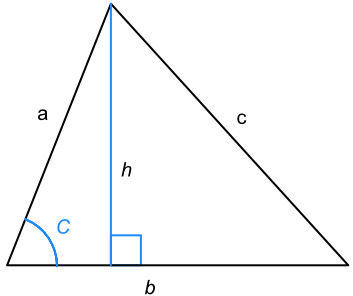Area of Any Triangle

## Area of Any Triangle

The area of a triangle is A = frac(1)(2)bh, where b is the base length and h is the vertical height.Using the trigonometrical ratios, the vertical height h = a xx sin C.

Substituting into the formula for the area of a triangle when the vertical height is known:

A = frac(1)(2) xx b xx h

A = frac(1)(2) xx b xx a xx sin C

A = frac(1)(2)ab sin C

## Example 1

What is the area of the triangle, below? Give your answer correct to 2 decimal places.Area of a triangle A = frac(1)(2)ab sin C substitute = frac(1)(2)(14)(19) sin (68) = 123.3155 to 2 dp = 123.32Area of a triangle A = frac(1)(2)ab sin C substitute 100 = frac(1)(2)(18)(20) sin x 100 = 180 sin x 0.55556 = sin x sin-1 both sides sin^-1(0.55556) = x 33.749 = x nearest degree 34 = x